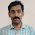Thursday, July 30, 2015

91-Magic Square Software (5 x 5) part-3

Third Part of Magic Square Software (5 x 5).
1) Click to read "Magic Square Software (5 x 5) part-1"
2) Click to read "Magic Square Software (5 x 5) part-2"
This is the third part of the 5x5 magic square software.

You can enter any 5 numbers between -9999 to 9999 (actually you can enter any number, but for the betterment of the look of the sheet, this restriction is implemented in the software) of your choice in the first row. The software will give you two different magic squares with your chosen numbers are in 1 st row. These two magic squares have 120 types of same addition.

 Rows 5 Columns 5 Diagonals 2 Broken Diagonals 8 Different Patterns 100 Total 120

Click the following button for the software of magic squares of order 5 x 5.

Now we will see all the properties of 5 x 5 magic squares one by one. Let us see the magic squares in which we choose the first row with the numbers as 9, 21, 34, 45 and 56. Following two magic squares will be obtained by the software.

Now in continuation of the previous blogs (Blog-99) and (Blog-100), we will see remaining types of addition:

In the previous blogs (99 and 100), we have studied the following points.

5) Addition of all the numbers in different pattern 01 : (21 to 45)

Here we define some names to the elements available in the cells of the magic square.
Let us define A11 as the element at 1st row 1st column. Similarly, the element at the 3rd row and 4th column will be defined as A34.

a) First element at First row Pattern A1 : (21-25/45)

Here, the addition of all the numbers placed at these places is the same and it is 165.

b) First element at Second-row Pattern A2 : (26-30/45)

Here, the addition of all the numbers placed at these places is the same and it is 165.

c) First element at Third-row Pattern A3 : (31-35/45)

Here, the addition of all the numbers placed at these places is the same and it is 165.

d) First element at Forth row Pattern A4 : (36-40/45)

Here, the addition of all the numbers placed at these places is the same and it is 165.

e) First element at Fifth row Pattern A5 : (40-45/45)

Here, the addition of all the numbers placed at these places is the same and it is 165.

6) Addition of all the numbers in different pattern 02 : (46 to 70)

c) First element at Third-row Pattern A3 : (56-60/70)

Here, the addition of all the numbers placed at these places is the same and it is 165.

d) First element at Forth row Pattern A4 : (61-65/70)

Here, the addition of all the numbers placed at these places is the same and it is 165.

e) First element at Fifth row Pattern A5 : (66-70/70)

Here, the addition of all the numbers placed at these places is the same and it is 165.

7) Addition of all the numbers in different pattern 03 : (71 to 95)

c) First element at Third-row Pattern A3 : (81-85/95)

Here, the addition of all the numbers placed at these places is the same and it is 165.

d) First element at Forth row Pattern A4 : (86-90/95)

Here, the addition of all the numbers placed at these places is the same and it is 165.

e) First element at Fifth row Pattern A5 : (91-95/95)

Here, the addition of all the numbers placed at these places is the same and it is 165.

8) Addition of all the numbers in different pattern 04 : (96 to 120)

c) First element at Third-row Pattern A3 : (106-110/120)

Here, the addition of all the numbers placed at these places is the same and it is 165.

d) First element at Forth row Pattern A4 : (111-115/120)

Here, the addition of all the numbers placed at these places is the same and it is 165.

e) First element at Fifth row Pattern A5 : (116-120/120)

Here, the addition of all the numbers placed at these places is the same and it is 165.

Now you can observe all the above 120 additions for your two magic squares prepared by using the following software. here their first rows will be of 5 numbers of your choice.

1.